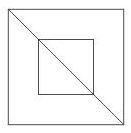Question 2

# How many triangles are there in the given figure ?Solution

Small triangles = EGH, EFH

Big triangles = ACD, ABD

Thus, total triangles = 4

=> Ans - (C)